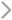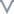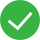DocumentationKingsoft Cloud Log ServiceUser GuideQuery syntaxSQL syntax

# SQL syntax

Last updated：2020-12-28 14:56:18

### Syntax

KLog supports basic SELECT queries in the following syntax:

``````    select_expr [, select_expr] ...
[WHERE where_condition]
[GROUP BY {col_name | expr}, ... ]
[ORDER BY {col_name | expr} [ASC | DESC], ...]
[LIMIT [offset,] row_count]

``````

### Operators and functions

#### Comparison functions

Operator Description
< Less than
> Greater than
<= Less than or equal to
>= Greater than or equal to
= Equal to
<> Not equal to
!= Not equal to
BETWEEN Determines whether a value is within a specified range.
IS NULL/IS NOT NULL Determines whether a value is NULL.

#### Logical operators

Operator Description
AND The result is true if both values are true.
OR The result is true if either value is true.
NOT The result is true if the value is false.

#### Arithmetic operators and functions

Operator or function Description
- Subtracts one value from another.
* Multiplies two values.
/ Divides one value by another to obtain a quotient.
% Divides one value by another to obtain a remainder.
abs(x) Returns the absolute value of x.
cbrt(x) Returns the cube root of x.
ceiling(x) Returns x rounded up to the nearest integer.
e() Returns Euler’s number.
exp(x) Returns Euler’s number raised to the power of x.
expm1(x) Returns exp(x) - 1.
floor(x) Returns x rounded down to the nearest integer.
log10(x) Returns the logarithm of x to base 10.
round(x) Returns x rounded to the nearest integer.
sqrt(x) Returns the square root of x.
acos(x) Returns the arc cosine of x.
asin(x) Returns the arc sine of x.
atan(x) Returns the arc tangent of x.
atan2(y,x) Returns the arc tangent of y/x.
cos(x) Returns the cosine of x.
sin(x) Returns the sine of x.
sinh(x) Returns the hyperbolic sine of x
cosh(x) Returns the hyperbolic cosine of x.
tan(x) Returns the tangent of x.

#### Date and time functions

Function Description
current_date() Returns the current date.
current_time() Returns the current time.
current_timestamp(date) Returns the current timestamp.
date_diff(expr,datetime1,datetime2) Returns the time difference in the specified unit between timestamp1 and timestamp2.
date_diff(expr,n,date) Returns the date and time after the time in the specified unit is added to the date.
datetime_format(datetime,expr) Returns the date and time in the format specified by expr.
datetime_parse(datetime,expr) Converts the date and time to the specified format.
time_parse(time,expr) Converts the time to the specified format.
date_part(datetime,expr) Extracts the specified part from the date and time.
date_trunc(datetime,expr) Sets the fields that are less than the specified value to zero in the date and time.
day_of_month(datetime) Extracts the day of the month from the date and time.
day_of_week(datetime) Extracts the day of the week from the date and time.
day_of_year(datetime) Extracts the day of the year from the date and time.
day_name(datetime) Extracts the day of the week from the date and time.
hour_of_day(datetime) Extracts the hour of the day from the date and time.
ios_day_of_week(datetime) Extracts the day of the week from the date and time.
ios_week_of_year(datetime) Extracts the week of the year from the date and time.
minute_of_day(datetime) Extracts the minute of the day from the date and time.
minute_of_hour(datetime) Extracts the minute of the hour from the date and time.
month_of_year(datetime) Extracts the month of the year from the date and time.
month_name(datetime) Extracts the name of the month from the date and time.
now() Returns the current timestamp.
second_of_minute(datetime) Extracts the second of the minute from the date and time.
quarter(datetime) Extracts the quarter from the date and time.
today() Returns the current date.
week_of_year(datetime) Extracts the week of the year from the date and time.
year(datetime) Extracts the year from the date and time.
extract(datetime_function from datetime) Extracts fields from the date and time by using the specified date and time function.

#### Aggregate functions

Function Description
avg(x) Returns the arithmetic average of x values.
count(*) Returns the number of rows.
count(distinct x) Returns the number of rows that have unique non-null x values.
count(all x) Returns the number of rows that have non-null x values.
first(a,b) Returns the first a value after data is sorted by b in the ascending order.
last(a,b) Returns the last a value after data is sorted by b in the ascending order.
max(x) Returns the maximum value.
min(x) Returns the minimum value.
sum(x) Returns the sum of x values.

#### Statistics functions

Function Description
kurtosis(x) Returns the distribution of x values.
mad(x) Returns the variability of x values.
kurtosis(x) Returns the distribution of x values.
percentile(x) Returns the nth percentile of x values.
percentile_rank(x) Returns the distribution of x values.
skewness(x) Returns the skewness of x.
stddev_pop(x) Returns the population standard deviation of x.
stddev_samp(x) Returns the sample standard deviation of x.
sum_of_squares(x) Returns the sum of squares of x.
var_pop(x) Returns the population variance of x.
var_samp(x) Returns the sample variance of x.

#### Loop function

Function Description
histogram(x,interval) Returns all matching values based on the specified interval.

#### Type conversion functions

Function Description
cast(x as data_type) Converts x to the specified data type.
Convert(x,data_type) Converts x to the specified data type.

Did you find the above information helpful?UnhelpfulMostly UnhelpfulA little helpfulHelpfulVery helpful

#### What might be the problems?

Insufficient
Outdated
Unclear or awkward
Redundant or clumsy
Lack of context for the complex system or functionality

#### More suggestions

0/200